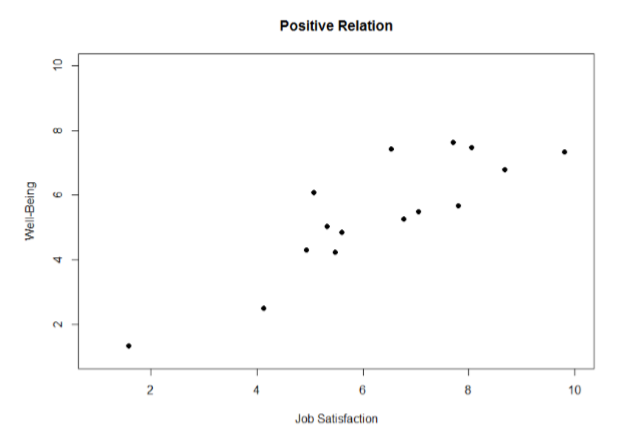# 13.2: Visualizing Relations

$$\newcommand{\vecs}{\overset { \rightharpoonup} {\mathbf{#1}} }$$ $$\newcommand{\vecd}{\overset{-\!-\!\rightharpoonup}{\vphantom{a}\smash {#1}}}$$$$\newcommand{\id}{\mathrm{id}}$$ $$\newcommand{\Span}{\mathrm{span}}$$ $$\newcommand{\kernel}{\mathrm{null}\,}$$ $$\newcommand{\range}{\mathrm{range}\,}$$ $$\newcommand{\RealPart}{\mathrm{Re}}$$ $$\newcommand{\ImaginaryPart}{\mathrm{Im}}$$ $$\newcommand{\Argument}{\mathrm{Arg}}$$ $$\newcommand{\norm}{\| #1 \|}$$ $$\newcommand{\inner}{\langle #1, #2 \rangle}$$ $$\newcommand{\Span}{\mathrm{span}}$$ $$\newcommand{\id}{\mathrm{id}}$$ $$\newcommand{\Span}{\mathrm{span}}$$ $$\newcommand{\kernel}{\mathrm{null}\,}$$ $$\newcommand{\range}{\mathrm{range}\,}$$ $$\newcommand{\RealPart}{\mathrm{Re}}$$ $$\newcommand{\ImaginaryPart}{\mathrm{Im}}$$ $$\newcommand{\Argument}{\mathrm{Arg}}$$ $$\newcommand{\norm}{\| #1 \|}$$ $$\newcommand{\inner}{\langle #1, #2 \rangle}$$ $$\newcommand{\Span}{\mathrm{span}}$$$$\newcommand{\AA}{\unicode[.8,0]{x212B}}$$

Chapter 2 covered many different forms of data visualization, and visualizing data remains an important first step in understanding and describing our data before we move into inferential statistics. Nowhere is this more important than in correlation. Correlations are visualized by a scatterplot, where our $$X$$ variable values are plotted on the $$X$$-axis, the $$Y$$ variable values are plotted on the $$Y$$-axis, and each point or marker in the plot represents a single person’s score on $$X$$ and $$Y$$. Figure $$\PageIndex{1}$$ shows a scatterplot for hypothetical scores on job satisfaction ($$X$$) and worker well-being ($$Y$$). We can see from the axes that each of these variables is measured on a 10-point scale, with 10 being the highest on both variables (high satisfaction and good health and well-being) and 1 being the lowest (dissatisfaction and poor health). When we look at this plot, we can see that the variables do seem to be related. The higher scores on job satisfaction tend to also be the higher scores on well-being, and the same is true of the lower scores.Figure $$\PageIndex{1}$$: Plotting satisfaction and well-being scores.

Figure $$\PageIndex{1}$$ demonstrates a positive relation. As scores on $$X$$ increase, scores on $$Y$$ also tend to increase. Although this is not a perfect relation (if it were, the points would form a single straight line), it is nonetheless very clearly positive. This is one of the key benefits to scatterplots: they make it very easy to see the direction of the relation. As another example, Figure $$\PageIndex{2}$$ shows a negative relation between job satisfaction ($$X$$) and burnout ($$Y$$). As we can see from this plot, higher scores on job satisfaction tend to correspond to lower scores on burnout, which is how stressed, un-energetic, and unhappy someone is at their job. As with Figure $$\PageIndex{1}$$, this is not a perfect relation, but it is still a clear one. As these figures show, points in a positive relation moves from the bottom left of the plot to the top right, and points in a negative relation move from the top left to the bottom right.Figure $$\PageIndex{2}$$: Plotting satisfaction and burnout scores.

Scatterplots can also indicate that there is no relation between the two variables. In these scatterplots (an example is shown below in Figure $$\PageIndex{3}$$ plotting job satisfaction and job performance) there is no interpretable shape or line in the scatterplot. The points appear randomly throughout the plot. If we tried to draw a straight line through these points, it would basically be flat. The low scores on job satisfaction have roughly the same scores on job performance as do the high scores on job satisfaction. Scores in the middle or average range of job satisfaction have some scores on job performance that are about equal to the high and low levels and some scores on job performance that are a little higher, but the overall picture is one of inconsistency.

As we can see, scatterplots are very useful for giving us an approximate idea of whether or not there is a relation between the two variables and, if there is, if that relation is positive or negative. They are also useful for another reason: they are the only way to determine one of the characteristics of correlations that are discussed next: form.Figure $$\PageIndex{3}$$: Plotting no relation between satisfaction and job performance.